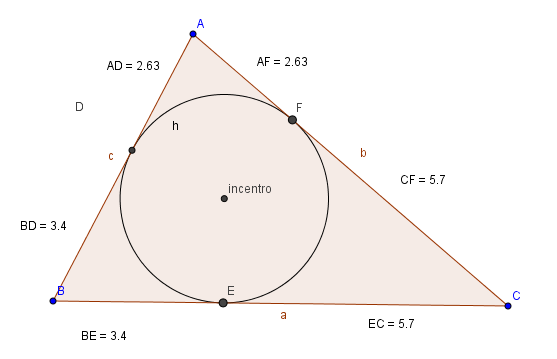Incenter

Incenter

The incenter of a triangle is the point where the internal angle bisectors of the triangle cross.

Constructing the Triangle Incenter

• Enable the tool POLYGON (Window 5) and click on three different places to form a triangle. In order to close the triangle click on the first point again. Naturally, the points cannot be aligned. A triangle with vertices at points A, B and C will be appear
• Enable the tool ANGLE BISECTOR (Window 4) and click on the vertices: A, C and B (in that order). Then click on the vertices C, B and A (in that order). Two bisectors were drawn with the names "d" and "e".
• Enable the tool INTERSECT TWO OBJECTS (Window 2). By intersecting lines “d” and “e”, point D will appear.
• We want to trace the third angle bisector. The question is, will this third bisector also passes through vertex D? Enable the tool ANGLE BISECTOR (Window 4) and click on the vertices: B, A and C (in that order).

Construction of the inscribed circle

In the previous app
• Enable the tool DISPLAY / HIDE OBJECT (Window 12), click on the lines d, e and f and then press ESC.
• Let us change the name of point D to Incenter. In order to do this, right click the mouse on point D and check the option RENAME. In the new window that will appear, type Incenter and click OK.
• Enable the tool Perpendicular Tool (Window 4), click on the Incenter point and on side c of the triangle (which connects points A and B).
• Enable the tool INTERSECT TWO OBJECTS (Window 2), click on line g and, then, on the side c (that connects points A and B). A vertex D will be appear .
• Our only interest is on the foot of the perpendicular (point D). Therefore, we can hide the line g, using the tool VIEW / HIDE OBJECT(Window 12). Having done that, you can press the ESC key.
• Enable the tool CIRCLE DEFINED BY THE CENTER AND ONE OF ITS POINTS (Window 6), click on the Incenter point and then on point D. A circle h will appear.

Analysis 1

Why do the sides of the triangle tangenciate the circle?

Analysis 2

Measure the distance from one of the vertices of the triangle to two points of tangency. Note that the measure of these distances are equal. Explain the reason why these measures are equal.Property

The three internal angle bisectors of a triangle intersect at the same equidist point on the 3 sides of the triangle.